Blog

# Bist-Parser : an end-to-end implementation of a Dependency Parser

Date: 2019-06-14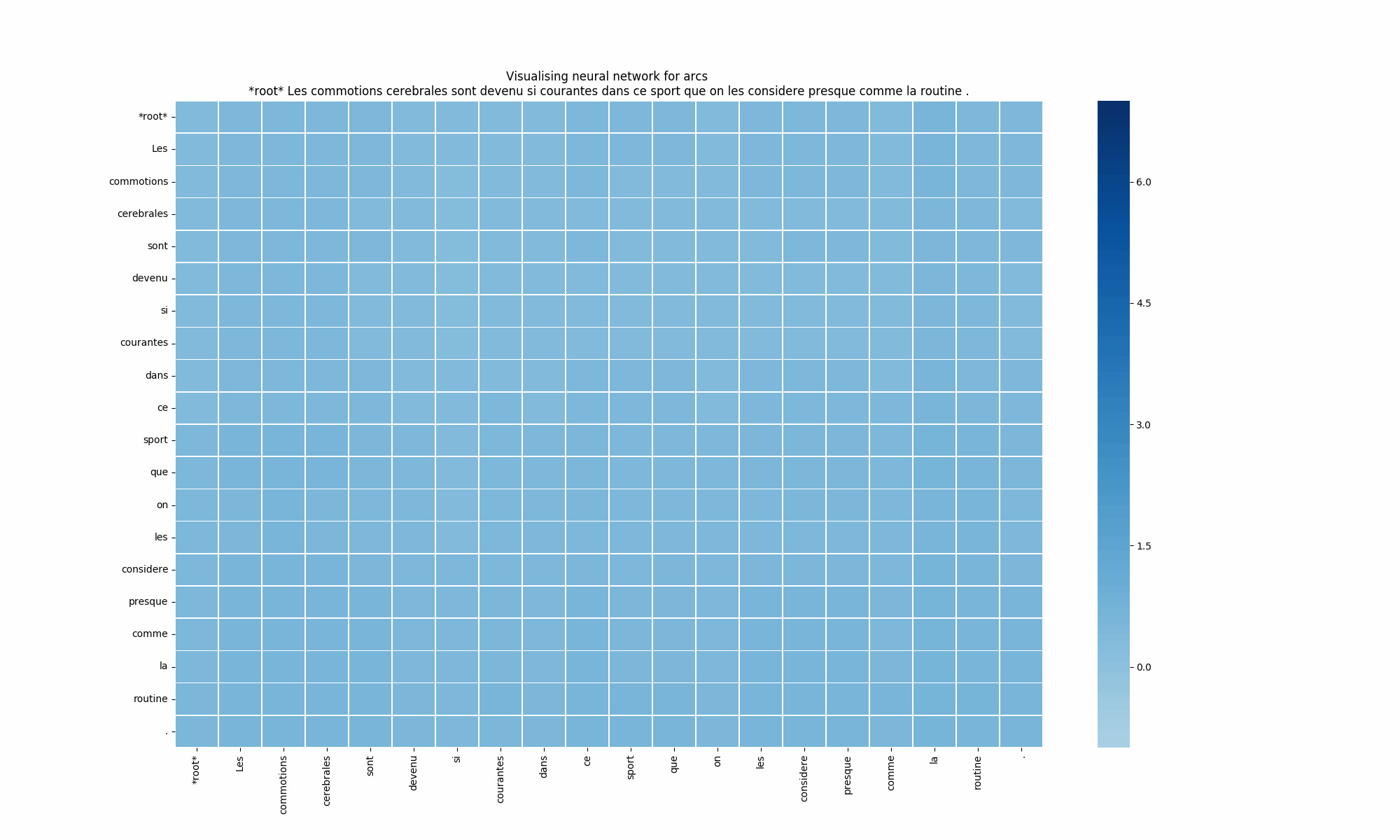## Vocabulary

• A TreeBank is a parsed text corpus that annotates syntactic or semantic sentence structure. Dependency TreeBanks are created using different approaches : either thanks to human annotators directly, or using automatic parsers to provide a first parse, then checked by annotators. A common approach consists in using a deterministic process to translate existing TreeBanks into new language through head rules. Producing a high-quality TreeBank is both time-consuming and expensive.

• CoNLL-U - Computational Natural Language Learning-Universal is a revised version of the CoNLL-X format. Sentences from TreeBanks are separated, and each word or punctuation mark is disposed on a distinct line. Each of the following items follows the word, separated by tabulations:

• ID: word index in the sentence, starting at 1

• FORM: word form or punctuation

• LEMMA: Lemma or stem of word form

• UPOS: Universal part of speech tag

• XPOS: Language-specific part of speech tag; will not be used in our model

• FEATS: Unordered list of morphological features, defined by Universal Dependencies; indicates the gender and number of a noun, the tense of a verb, etc.

• HEAD: Head of the word, indicates the index of the word to which the current one is related

• DEPREL: Universal Dependencies relation; indicates the relation between two words (subject or object of a verb, determiner of a noun, etc.)

• DEPS: Language-specific part of speech tags; will not be used in our model

• MISC: Commentary or other annotation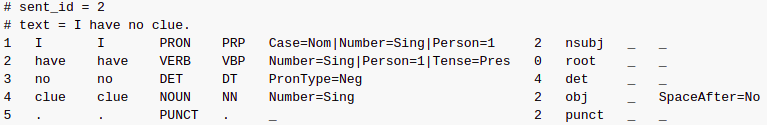An example of CoNLL-U format

• An Entry is a word, or a punctuation mark in a sentence. It has multiple attributes, defined above. A sentence is typically a concatenation of entries (a word itself is an attribute of an entry: its form), separated by space.

## The Implementation

The implementation of the Bist-Parser comes from the authors of its paper. An update has been published on GitHub by Xiezhq Hermann. You can find it here. It works on Python 3.x, with torch 0.3.1 (with or without Cuda). It is very complete and can be used as is. However, in order to adapt the code to your data or upgrade it, you must get through every module, which can be a difficult task. This part of the article will lead you through all files and processes.

Universal Dependencies (UD) is an open-community framework for grammatical annotation. It provides corpora and tools that greatly help to develop a Dependency Parser.

From UD, you can download a corpus of sentences of your choice (in any language available, even Old French!), use them as is, and start training your Bist-Parser with this type of command:

python src/parser.py --outdir `[`results directory`]` --train training.conll --dev development.conll --epochs 30 --lstmdims 125 --lstmlayers 2 `[`--extrn extrn.vectors`]`

You can detail hyperparameters here, caught by the model thanks to the file parser.py

As you may know, when you train a model on a corpus, the model is biased towards this corpus. You could train your model on multiple corpora in order to generalize it more. Several techniques allow you to increase scores, with TreeBank Embedding as an example. Here, we have just concatenated some TreeBanks, without any further processing.

### utils

• Create a ConllEntry class: every entry has well-known attributes: id, form, lemma, Universal PoS tag, language Specific PoS tag, morphological features, head of current word, dependency relation, enhanced dependency relation and commentary. These attributes are defined from the Universal Dependencies CoNLL-U format. This format is useful for the model to understand what its inputs are, and what it should predict.

• Read a CoNLL-U file and transform each sentence into a ConllEntry.

• Count vocabulary: This function creates a Counter of ConllEntry attributes and allows you to know how these attributes are distributed through your dataset. If you want to determine the most frequent words or relations in your dataset, this function can be useful.

mstlstm

This file contains your model. All your hyper-parameters and most of your monitoring work happen in this file.

The method forward iterates through each entry in the sentence. It first computes the vectors for each entry attribute. With our model, we get multiple vectors that describe the word, the PoS tag and the feats. Those vectors are then concatenated to form a vector with a bigger dimension for each entry. These entries are then concatenated together to form the sentence vector.

``````def forward(self, sentence, errs, lerrs):
for entry in sentence:
c = float(self.wordsCount.get(entry.norm, 0))
# dropFlag = (random.random() < (c / (0.33 + c)))
dropFlag = (random.random() < (c / (0.25 + c)))
wordvec = self.wlookup(scalar(
int(self.vocab.get(entry.norm, 0)) if dropFlag else 0)) if self.wdims > 0 else None
ontovec = self.olookup(scalar(int(self.onto[entry.onto]) if random.random(
) < 0.9 else 0)) if self.odims > 0 else None
cposvec = self.clookup(scalar(int(self.cpos[entry.cpos]) if random.random(
) < 0.9 else 0)) if self.cdims > 0 else None
posvec = self.plookup(
scalar(int(self.pos[entry.pos]))) if self.pdims > 0 else None
# posvec = self.plookup(
#     scalar(0 if dropFlag and random.random() < 0.1 else int(self.pos[entry.pos]))) if self.pdims > 0 else None
evec = None
if self.external_embedding is not None:
evec = self.elookup(scalar(self.extrnd.get(entry.form, self.extrnd.get(entry.norm, 0)) if (
dropFlag or (random.random() < 0.5)) else 0))
entry.vec = cat([wordvec, posvec, ontovec, cposvec, evec])
entry.lstms = [entry.vec, entry.vec]
entry.modfov = None
entry.rmodfov = None
num_vec = len(sentence)
vec_for = torch.cat(
[entry.vec for entry in sentence]).view(num_vec, 1, -1)
vec_back = torch.cat(
[entry.vec for entry in reversed(sentence)]).view(num_vec, 1, -1)
res_for_1, self.hid_for_1 = self.lstm_for_1(vec_for, self.hid_for_1)
res_back_1, self.hid_back_1 = self.lstm_back_1(
vec_back, self.hid_back_1)
vec_cat = [cat([res_for_1[i], res_back_1[num_vec - i - 1]])
for i in range(num_vec)]
vec_for_2 = torch.cat(vec_cat).view(num_vec, 1, -1)
vec_back_2 = torch.cat(list(reversed(vec_cat))).view(num_vec, 1, -1)
res_for_2, self.hid_for_2 = self.lstm_for_2(vec_for_2, self.hid_for_2)
res_back_2, self.hid_back_2 = self.lstm_back_2(
vec_back_2, self.hid_back_2)
for i in range(num_vec):
sentence[i].lstms = res_for_2[i]
sentence[i].lstms = res_back_2[num_vec - i - 1]
scores, exprs = self.__evaluate(sentence, True)
gold = [entry.parent_id for entry in sentence]
rscores, rexprs = self.__evaluateLabel(
goldLabelInd = self.rels[sentence[modifier + 1].relation]
wrongLabelInd = \
max(((l, scr) for l, scr in enumerate(rscores)
if l != goldLabelInd), key=itemgetter(1))
if rscores[goldLabelInd] < rscores[wrongLabelInd] + 1:
lerrs += [rexprs[wrongLabelInd] - rexprs[goldLabelInd]]
e = sum([1 for h, g in zip(heads[1:], gold[1:]) if h != g])
if e > 0:
errs += [(exprs[h][i] - exprs[g][i])
for i, (h, g) in enumerate(zip(heads, gold)) if h != g]
return e
``````

First, it converts entries into vectors. Here, the principal attributes are the embedding of words, lemmas (onto) and PoS tags (pos). However, we advise you to add as many features as possible. For example, you may have access to features of words that indicate whether the noun is singular or plural, its gender, or tense... Embedding these features allows your BiLSTM to find many more patterns.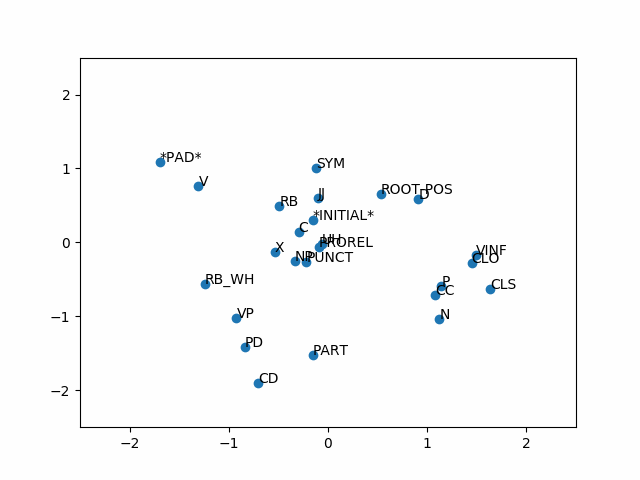Evolution of PoS embedding on two dimensions

Then, it feeds the BiLSTM with these vectors (for = forward, back = backward). Line 52 evaluates the scores of the sentence. This is the part where the full Weighted Digraph is created. On line 57, it evaluates the relation score. This is an interesting trick in this model: rather than evaluating all the possibilities at the same time (|possibilities|=|arcs|.|labels|, which is way too high), it predicts the dependencies first, then the relations.

We will see about errs,* lerrs *and e later.

In the illustrations below, you can see the evolution of dependency evaluation through batches. A dark blue cell corresponds to a weighted arc. The example comes from a typical French sentence, "Les commotions cérébrales sont devenu si courantes dans ce sport qu'on les considère presque comme la routine." You can spot spelling mistakes in the sentence; this is not rare in TreeBanks.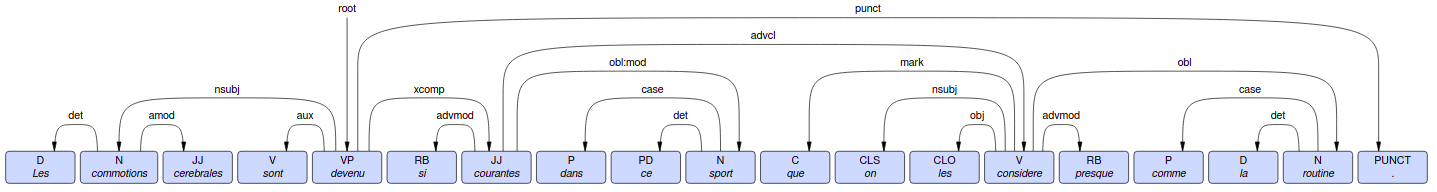Les commotions cérébrales sont devenu si courantes dans ce sport qu'on les considére presque comme la routine.

Noteworthy points in this sentence are the word, "devenu," which is the root (i.e. the main word), and the three clearly defined propositions separated by the words, "que" and "comme."Evolution of dependency scores for the sentence "root Les commotions cerebrales sont devenu si courantes dans ce sport que on les considère presque comme la routine."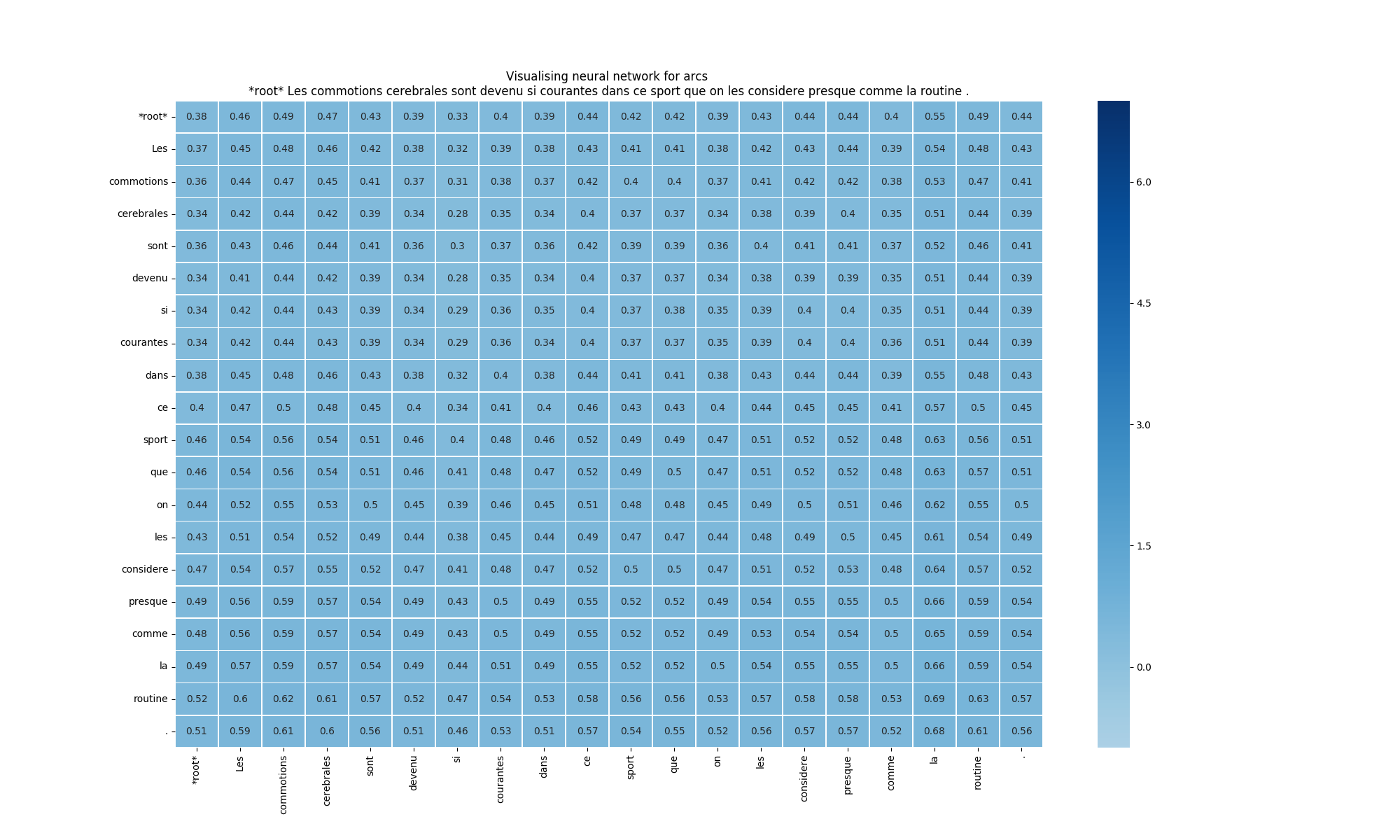Scores figure (1) - Initialization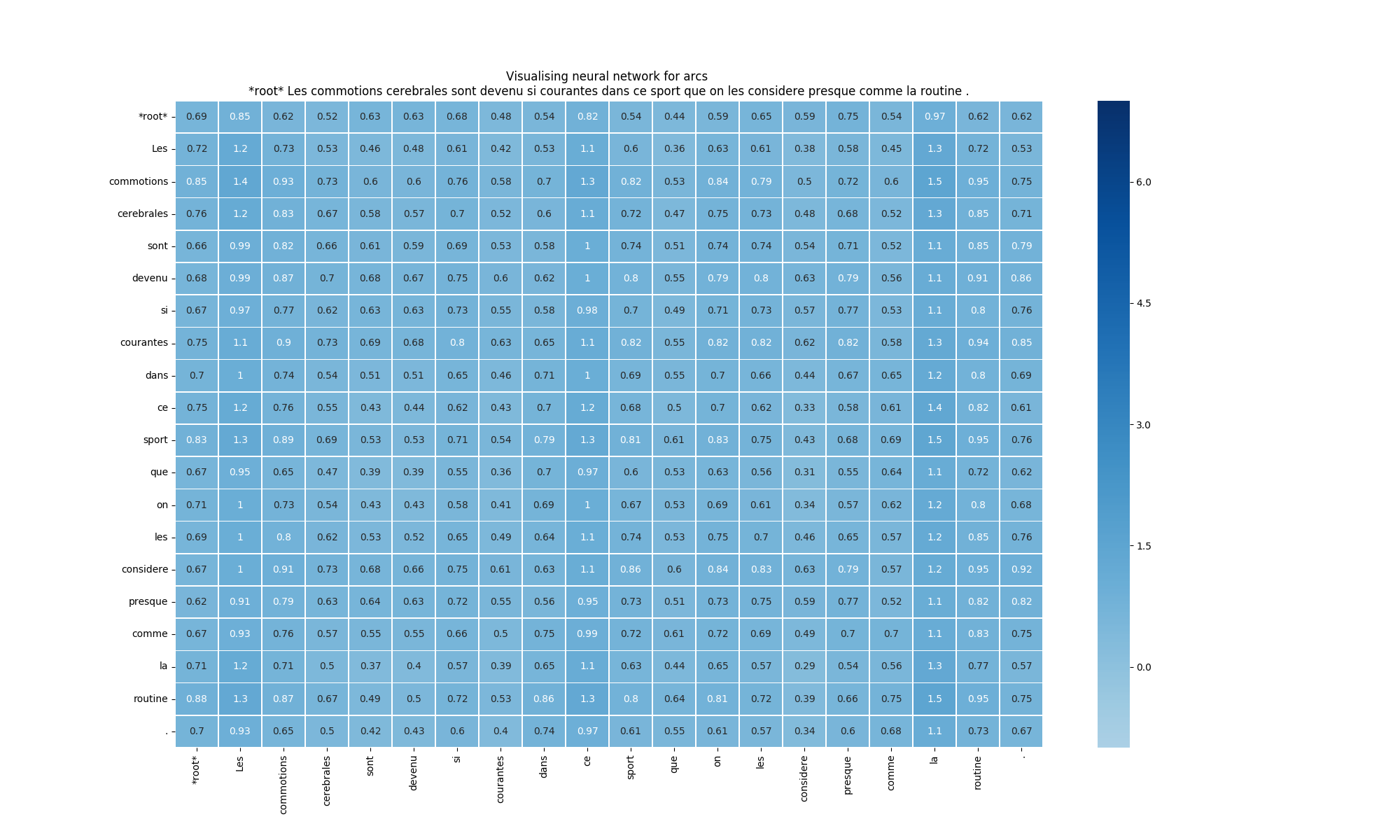Scores figure (2) - 200 sentences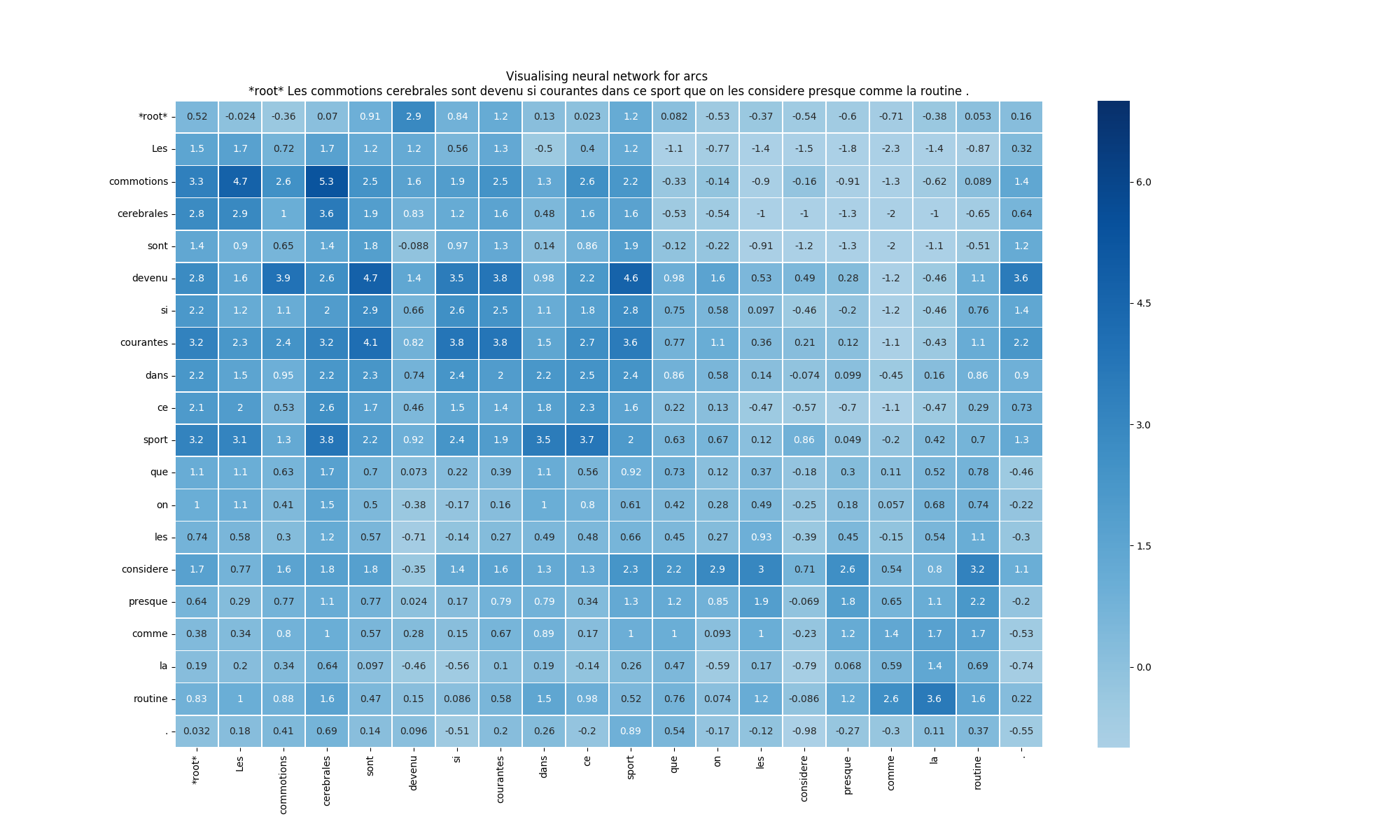Scores figure (3) - 1000 sentences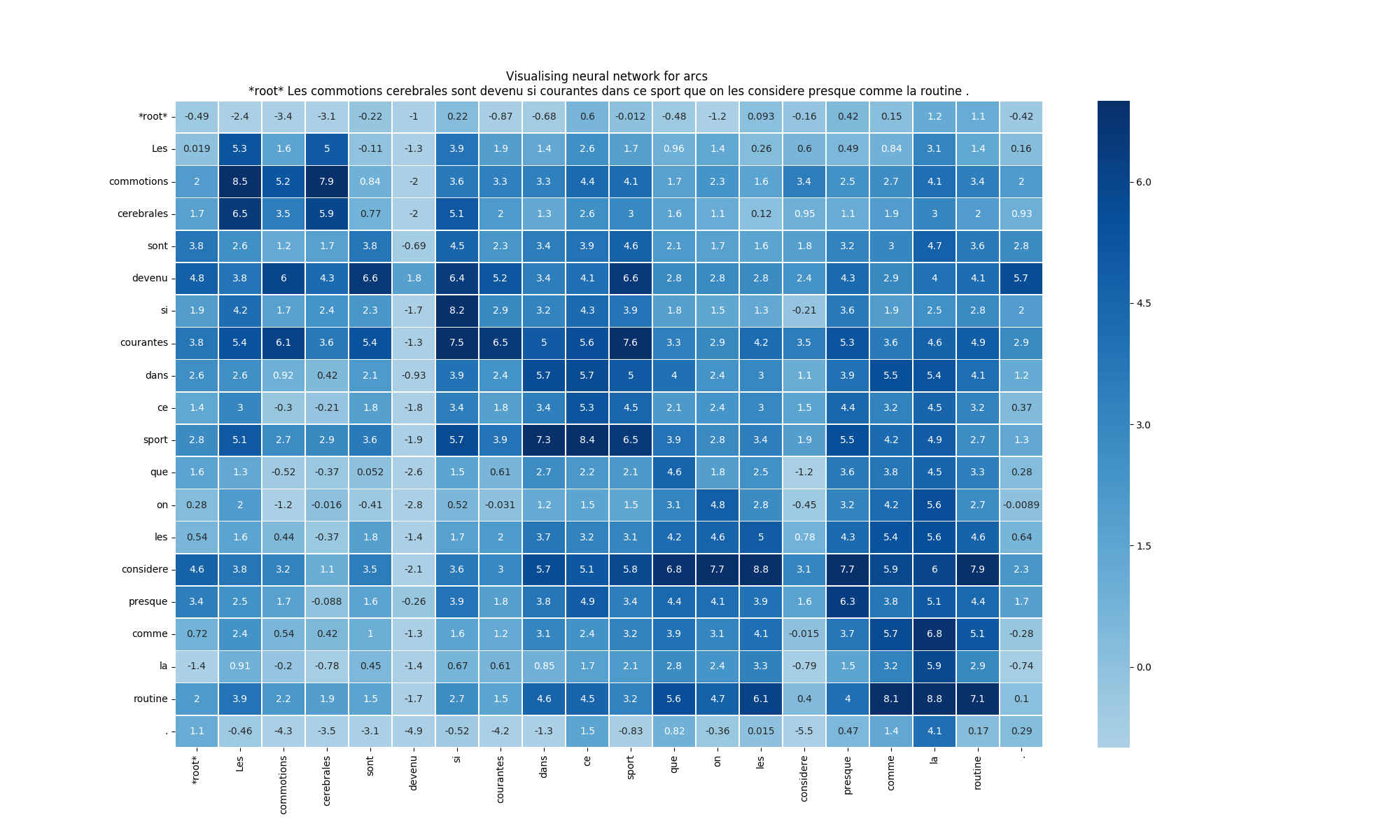Score figure (4) - 6000 sentences

In the above illustrations, we can better understand the evolution of our neural network. Each column corresponds to a token as head, each line corresponds to a token as dependent, and each cell corresponds to the score (or weight) of the arc from head to dependent.

We recognize the random initialization in figure 1, where all the scores are around zero, and we cannot see any shape in the matrix.

In the second picture, we can see that PoS=Determinant vector has been taken into account, and we can make out a shape in their columns. Changes along rows are less visible for the moment.

In the third illustration, we can clearly spot the two propositions, separated by "que." Arcs are well defined before it, and a bit less so after it. The punctuation mark is well linked to the root "devenu."

In the last one, we have a clear idea of principle arcs, the model gains confidence, and score values are more outspread.

Once dependencies are predicted, the model predicts the relation type. Here are plots of the scores given for each relation, regarding the predicted relation, given the correct dependency.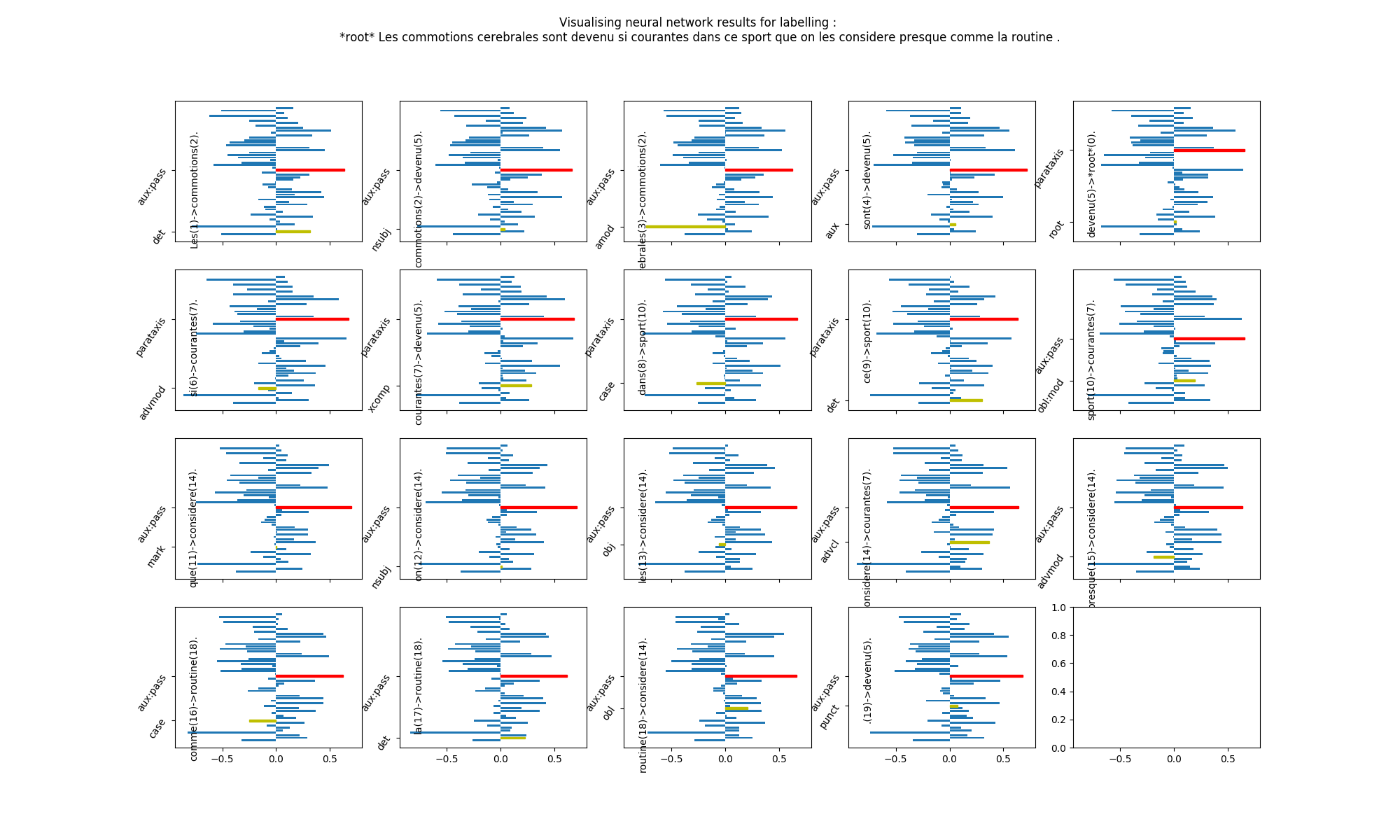Relation score figure (1) - Initialization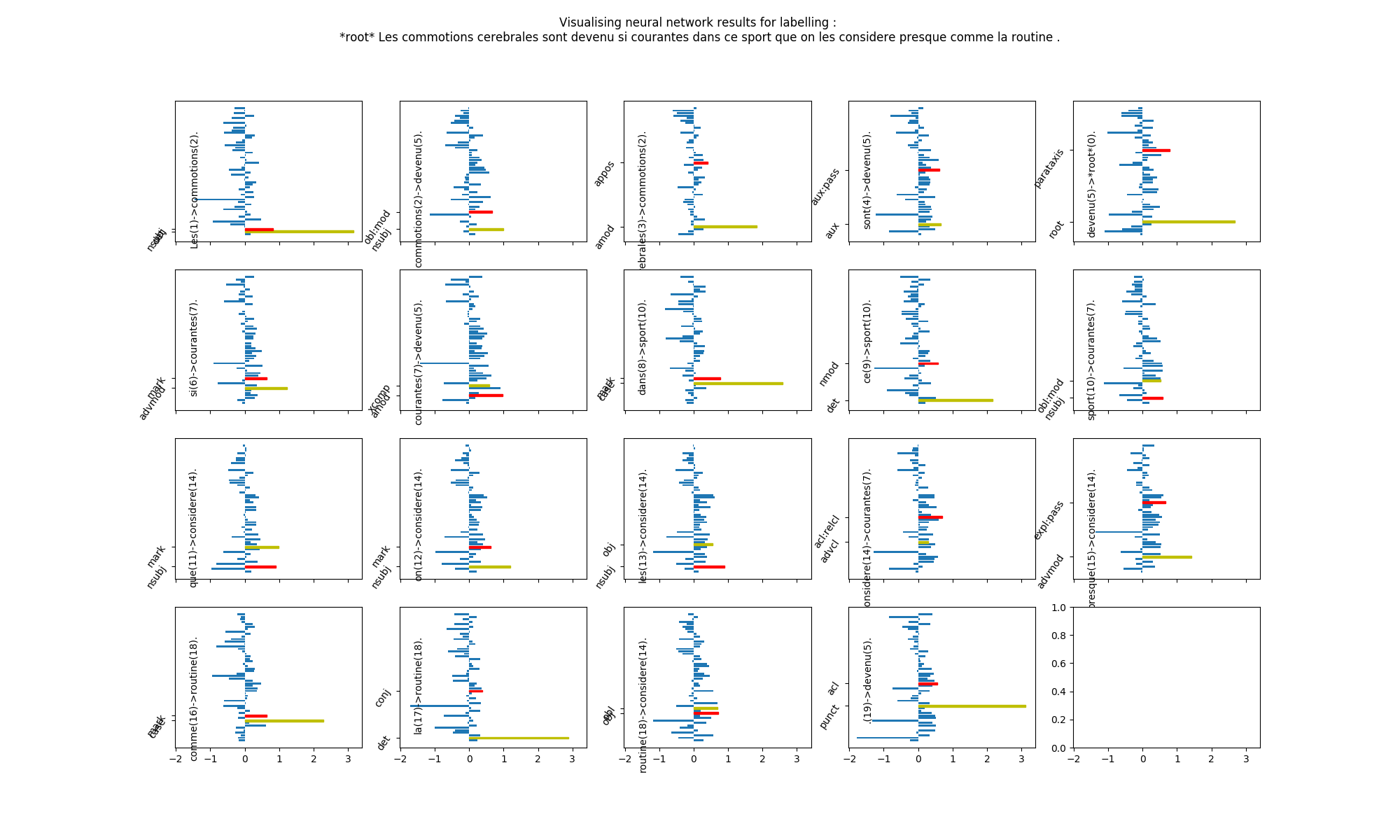Relation score figure (2) - 200 sentences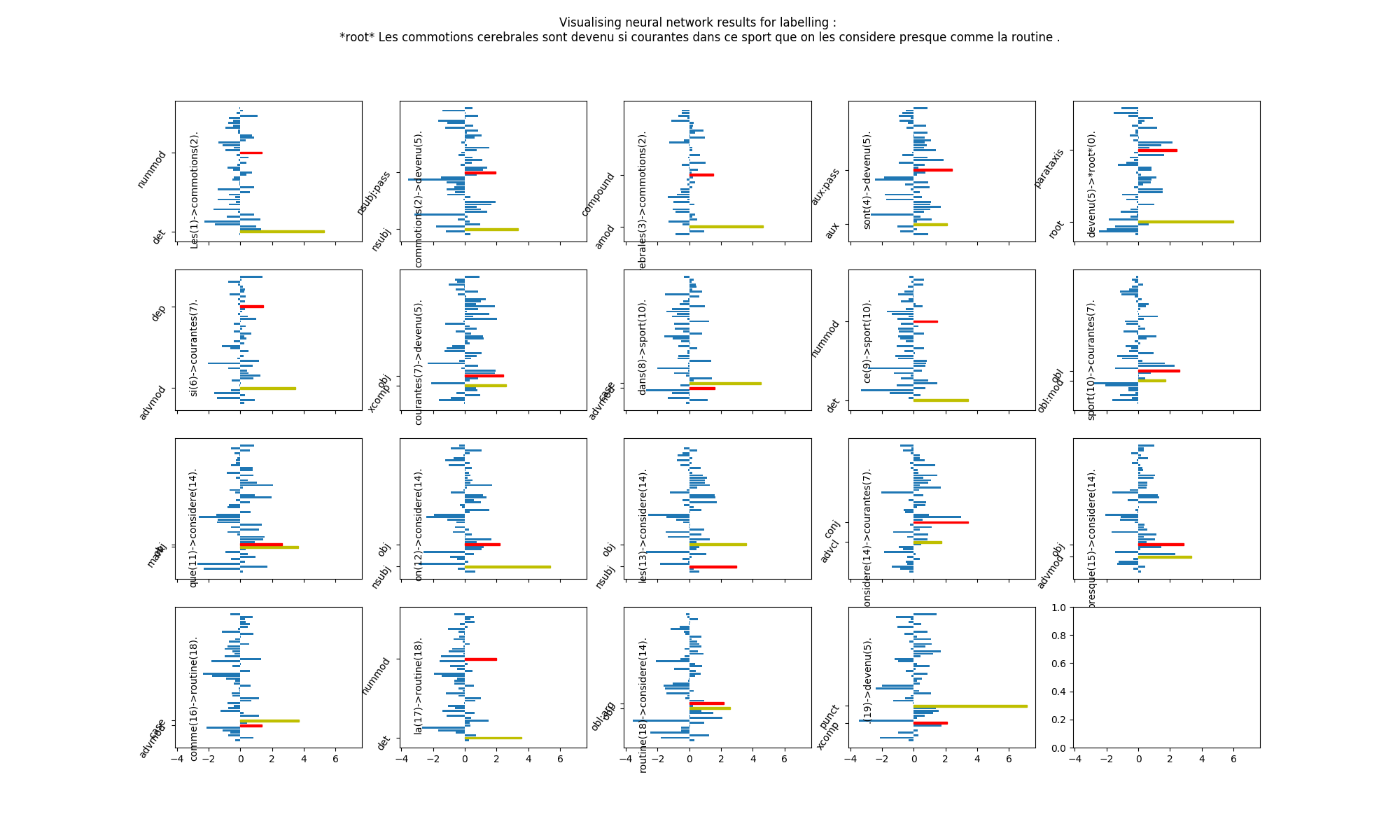Relation score figure (3) - 1000 sentences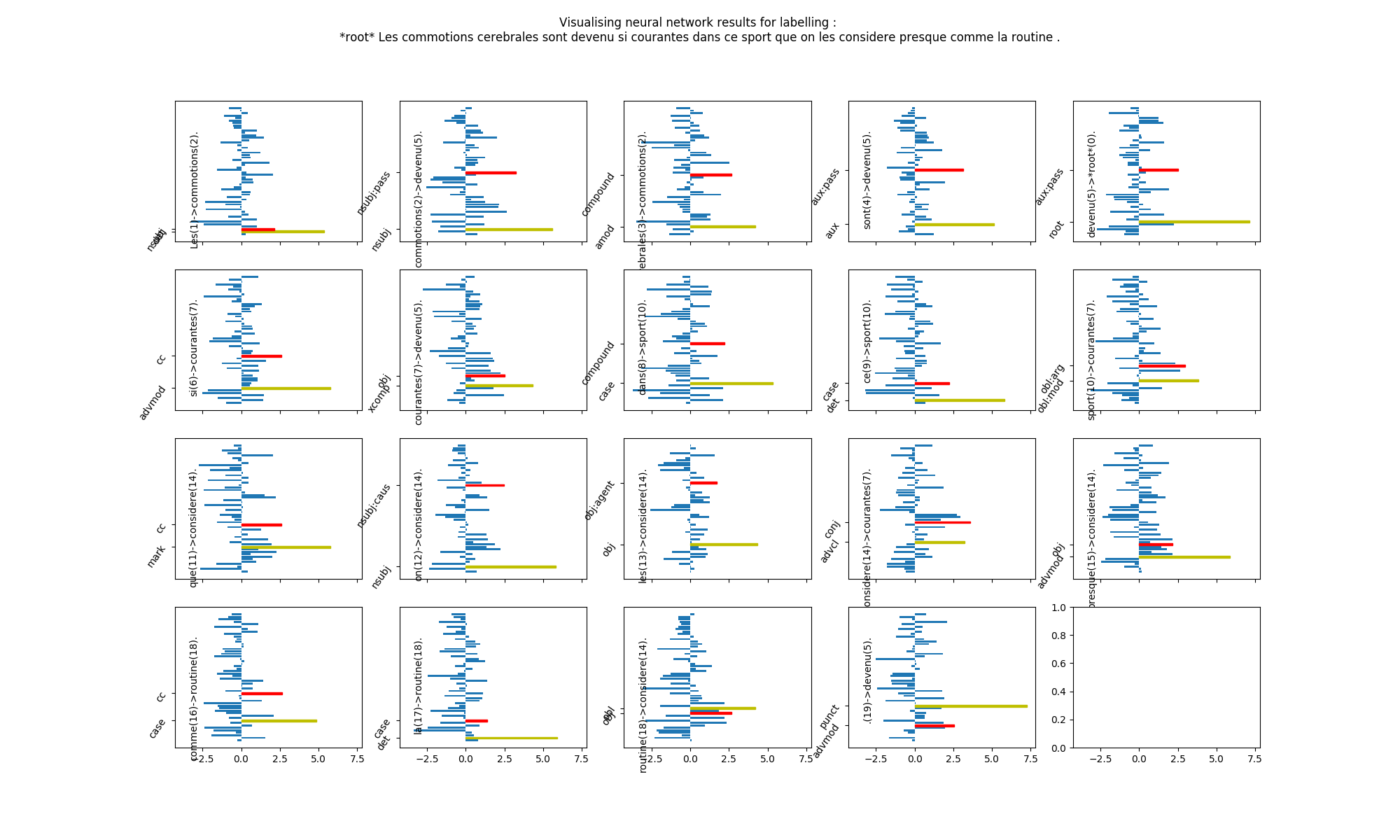Relation score figure (4) - 6000 sentences

The relation type predicted is in yellow and the highest incorrect one is in red.

After some training, the prediction becomes more and more confident.

``````def train(self, conll_path):
print('pytorch version:', torch.__version__)
batch = 1
eloss = 0.0
mloss = 0.0
eerrors = 0
etotal = 0
iSentence = 0
start = time.time()
with open(conll_path, 'r') as conllFP:
random.shuffle(shuffledData)
errs = []
lerrs = []
for iSentence, sentence in enumerate(shuffledData):
self.model.hid_for_1, self.model.hid_back_1, self.model.hid_for_2, self.model.hid_back_2 = [
self.model.init_hidden(self.model.ldims) for _ in range(4)]
if iSentence % 100 == 0 and iSentence != 0:
print('Processing sentence number:', iSentence,
'Loss:', eloss / etotal,
'Errors:', (float(eerrors)) / etotal,
'Time', time.time() - start)
start = time.time()
eerrors = 0
eloss = 0.0
etotal = 0

conll_sentence = [entry for entry in sentence if isinstance(
entry, utils.ConllEntry)]
e = self.model.forward(conll_sentence, errs, lerrs)
eerrors += e
eloss += e
mloss += e
etotal += len(sentence)
if iSentence % batch == 0 or len(errs) > 0 or len(lerrs) > 0:
if len(errs) > 0 or len(lerrs) > 0:
eerrs = torch.sum(cat(errs + lerrs))
eerrs.backward()
self.trainer.step()
errs = []
lerrs = []
if len(errs) > 0:
eerrs = (torch.sum(errs + lerrs))
eerrs.backward()
self.trainer.step()
print("Loss: ", mloss / iSentence)
``````

The main method of the model is train. It first shuffles sentences to train the model with a different order each time. Then, the method forward is called for each sentence. This will update weights, return a score e and update two lists errs and lerrs. Recall that e counts each token for which the predicted head is different than the gold head (the reference, from the corpus). *errs *computes the loss on arcs prediction, and lerrs computes the loss on labels prediction. errs and lerrs are then summed to produce the backpropagation loss: eerrs.

## Improvements and a few results

In order to make an even more efficient model, you should try to add word dropout as an hyperparameter, and try various values. We have tried 0, 0.25, 0.33, 0.5, 0.9 and 1 as values, and the best one was 0.9. It enforces the model to learn a lot more from other vectors than words.

You can also separate each feature and use embedding on each of them. This would allow more flexibility and more understanding from the model.

With such implementation, we have obtained a **LAS score of 87.70 **on French Sequoia (Universal Dependencies) TreeBank, without training specifically on this corpus (after tag translation, since our tags are different than UD's).

Once your Dependency Parser is trained, you can use dependencies and relations to have a better understanding of your data. Even if a NLU model is able to find many patterns, adding these inputs can be a real gain that you should consider.